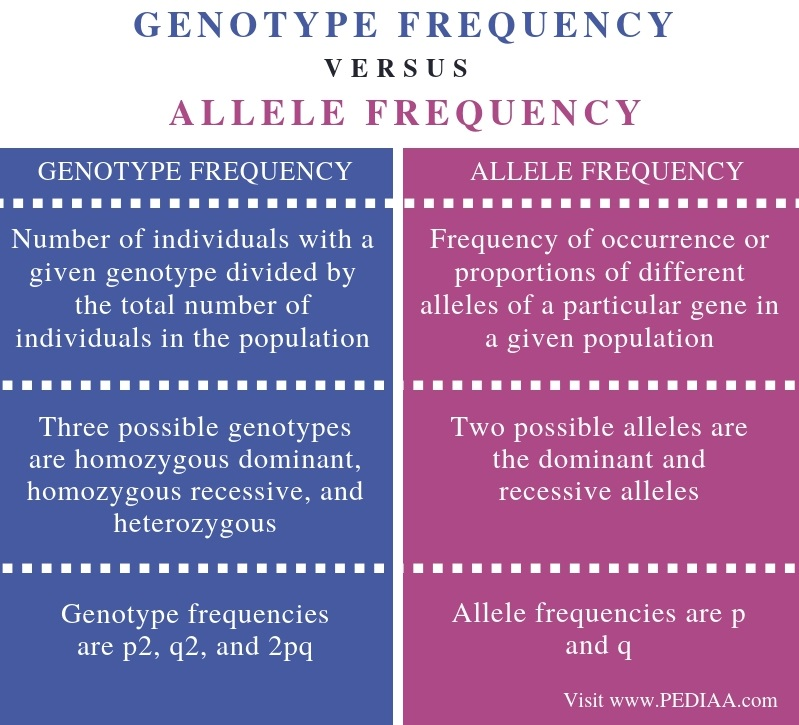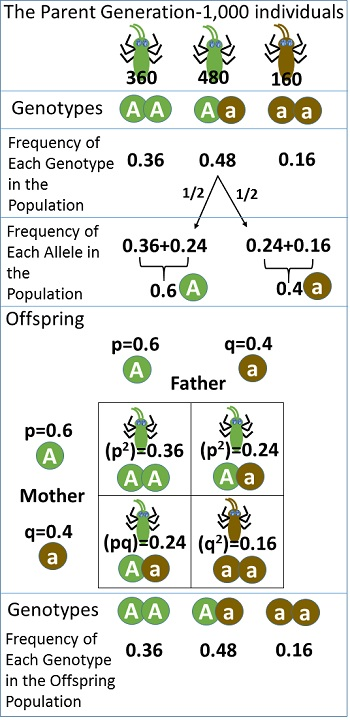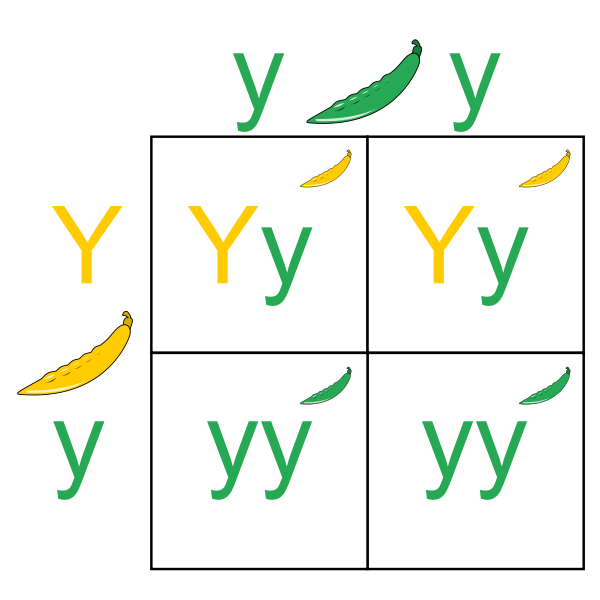# What is the Difference Between Genotype Frequency and Allele Frequency

The main difference between genotype frequency and allele frequency is that the genotype frequency is the frequency of the possible three genotypes in a population: homozygous dominant (AA), homozygous recessive (aa), and heterozygous (Aa) whereas the allele frequency is the frequency of the two types of alleles in a population: dominant (A) and recessive (a) alleles. Furthermore, the p2, q2, and 2pq values represent the three possible genotypes while p and q represent the two alleles in the population respectively.

Genotype frequency and allele frequency are two parameters used in the Hardy-Weinberg equation. This equation calculates the genetic variation of a population at equilibrium.

### Key Areas Covered

1. What is Genotype Frequency
– Definition, Possible Genotypes, Equation
2. What is Allele Frequency
– Definition, Possible Genotypes, Equation
3. What are the Similarities Between Genotype Frequency and Allele Frequency
– Outline of Common Features
4. What is the Difference Between Genotype Frequency and Allele Frequency
– Comparison of Key Differences

### Key Terms

Allele Frequency, Genotype Frequency, Genetic Variation, Hardy-Weinberg Equation, Heterozygous, Homozygous## What is Genotype Frequency

Genotype frequency is the frequency of the three possible genotypes which can occur in a particular population. They are the homozygous dominant, homozygous recessive, and the heterozygous genotypes. Each frequency of the genotype can be calculated by dividing the number of individuals that show a particular genotype by the total number of individuals in the population.Figure 1: Genotypic Frequency and Allele Frequency in the Hardy-Weinberg Equation

Hence, the final Hardy-Weinberg equation becomes;

p2 + 2pq + q2 = 1

Here, the p2 value represents the homozygous dominant frequency, and the q2 represents the homozygous recessive frequency while the 2pq value represents the heterozygous genotype frequency.

## What is Allele Frequency

The allele frequency is the frequency of the two forms of a particular allele in a population. They are dominant and recessive alleles. Each allele frequency can be calculated by dividing the number of individuals with the allele form by the total number of individuals in the population. Here, the p represents the dominant allele frequency of the population while the q allele represents the recessive allele frequency. Also, the sum of the allele frequencies in a population is equal to 1.

p + q = 1Figure 2: Inheritance of Dominant and Recessive Alleles

## Similarities Between Genotype Frequency and Allele Frequency

• Genotype frequency and allele frequency are two types of parameters that describe the genetic variation of a population in the Hardy-Weinberg equation.
• The sum of all possible frequencies is equal to 1.

## Difference Between Genotype Frequency and Allele Frequency

### Definition

Genotype frequency refers to the number of individuals with a given genotype divided by the total number of individuals in the population while allele frequency refers to the frequency of occurrence or proportions of different alleles of a particular gene in a given population. Thus, this is the main difference between genotype frequency and allele frequency.

### Components

The three possible genotypes are the homozygous dominant, homozygous recessive, and heterozygous while the two possible alleles are the dominant and recessive alleles.

### In Hardy-Weinberg Equation

The genotype frequencies are p2, q2, and 2pq values while allele frequencies are p and q. Hence, the type of constituent frequencies is a major difference between genotype frequency and allele frequency.

### Conclusion

Genotype frequency is the frequency of each of the three possible genotypes in the population. Where, the three genotypes are the homozygous dominant, homozygous recessive, and the heterozygous genotypes. On the other hand, the allele frequency is the frequency of the two possible forms of alleles in the population, which are the dominant and recessive alleles. However, both genotype frequency and allele frequency are important for the calculation of genetic variation of a population according to Hardy-Weinberg equation. In brief, the main difference between genotype frequency and allele frequency is the type of constituent frequencies.

##### Reference:

1. “Hardy-Weinberg Equation.” Nature News, Nature Publishing Group, Available Here

##### Image Courtesy:

1. “Hartsock Hardy Weinberg Example” By Angelahartsock – Own work (CC0) via Commons Wikimedia
2. “Punnett Square” By Pbroks13 – Own work (CC BY-SA 3.0) via Commons Wikimedia